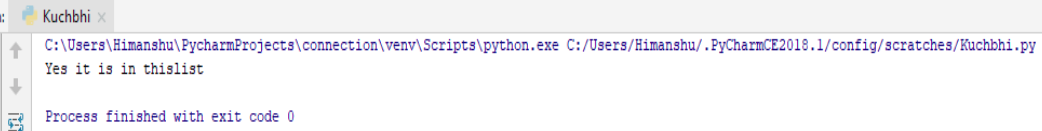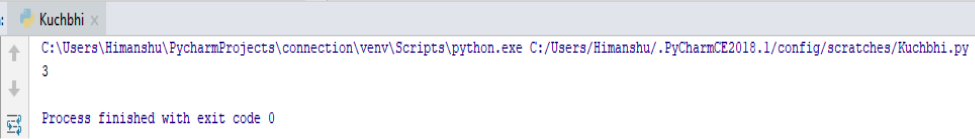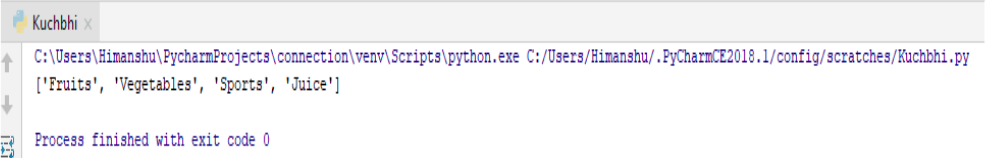×

### Html & Html5

Introduction to Html

Html5 Grid

Html5 Graphics

Html5 Media

### Python List

A list is a collection which is ordered and changeables. In Python lists are written with square brackets [].
Examples:-

Create a list

thislist =["Fruits", "Vegetables", "Sports"]

print(thislist)

### Output of this Program

So here in above program we had given the command of creating List to our Python interpreter. Let’s see .So our List is been ready.

### Access Items in List

Here in the List data type , if we want to access any particular items from the list , than we can write the index number of specified element inside the square bracket.

Let’s understand this by an example :-

thislist = ["Fruits", "Vegetables", "Sports"]

print(thislist)

### Output of this Program

So here in the above program we just want to access only one item from the list who is having index no. 2, means ‘Sports’ , we want because first one is included as index or number ‘0’ in Python.
So let’s see what output it gives :-Here it just show us the desired item which we want.

### Change Item Value in List

We can not only access the items in the List but although we can also change the item value, just by giving reference of the index number.

Let’s understand this by an example :-

thislist = ["Fruits", "Vegetables", "Sports"]

thislist= "Juice"

print(thislist)

### Output of this Program

So here in the above Program we just gave command to our Python interpreter that we want to change the item , of index number ‘1’ from ‘Vegetables’ to ‘Juice’ .
So let’s see what output it gives :-Here it just show us the desired item which we want.

### Loops through List

As we all know that loops are the repetition of the same ‘string’ , ‘item’ or etc. under the same condition . In Python we can loop through the list by using the ‘for’ loop .

Let’s understand this by an example :-

thislist = ["Fruits", "Vegetables", "Sports"]

for x in thislist :

print(x)

### Output of this Program

So here in the above program we just gave command to our Python interpreter that we want to print all the items , one by one .
So let’s see what output it gives :-Here it just shows all the items one by one.

( More about Loops we will talk further on the looping section. )

### Check if item exist in List

We can also check items or elements in our list by using the ‘in’ keyword.
Let us understand this by the following example :-

thislist = ['Fruits', 'Vegetables', 'Sports']

if 'Vegetables' in thislist :

print("Yes it is in thislist")

'

### Output of this ProgramSo our ‘Vegetable’ is in the list . So it just give the correct output.

( We will read in detail regarding ‘if’ statement . )

### Methods in List

As we know that methods are basically the function which we want to perform in our coding . In Python there are some inbuilt methods are there for List too .
So let’s see some of them :-

The len() method - It just returns the length of the list , base on their index number

thislist = ['Fruits', 'Vegetables', 'Sports']

print(len(thislist))

### Output of this ProgramSo here we just get the length of the list based on their index number.

Adding items on the List - the append() method is used to add items in the List

thislist = ['Fruits', 'Vegetables', 'Sports']

thislist.append ("Juice")

print(thislist)

### Output of this ProgramHere item ‘Juice’ also included just by the method of append ()

Clear items from the List - the clear() method is used to clear the items from the list:-

thislist = ['Fruits', 'Vegetables', 'Sports']

thislist.clear ()

print(thislist)

### Output of this ProgramSo here through clear() method all the items or elements are gone.

### Some methods of List and their functionality

So here we are enlisting some methods of list and their description which we can use in Python.

Method Description
append() Adds the elements of end of the list.
clear() Remove all elements from the list.
copy() Returns a copy of the lists.
count() Return the number of element with the specified values.
extends() Add the elements of list,to the end of the current list.
index() Returns the index of the first element with the specified value
insert() Adds an elements at the specified position.
pop() Removes an elements at the specified position.
remove() Removes an item at the specified value.
reverse() Reverse the order of the list.
sort() Sorts the list.

Check all these methods for good practice.

python training insitute| Best IT Training classes in Gurgaon | python training